Chapter 7: Inertia

# 7.2 Center of Mass: Composite Shapes

Instead of integrating to find the center of mass, we can split an object up into recognizable shapes to determine the center of mass. This is faster than the integration method, and allows for many different ways to find the answer.

In this section, the terms ‘centroid’ and ‘center of mass’ are used, somewhat interchangeably. The centroid is the geometric center. The center of mass takes into account the density of an object. If the density is the same throughout an object, then the centroid is the same as the center of mass. For the rigid beams we will be looking at in statics, the centroid is at the same location as the center of mass.

# 7.2.1 Centroid Tables

We use the centroid tables that are listed below to combine the locations of the centers of mass for each shape. Approximations are needed as real life objects are rarely perfectly square or circular, but if they are symmetric, it makes it easier to approximate.

The locations of the center of mass (rcm) are as follows. The source for the images are from Jacob Moore et al. http://mechanicsmap.psu.edu/websites/centroidtables/centroids2D/centroids2D.html

 Shape Images from Jacob Moore et al. http://mechanicsmap.psu.edu/websites/centroidtables/centroids2D/centroids2D.html rcm Rectangle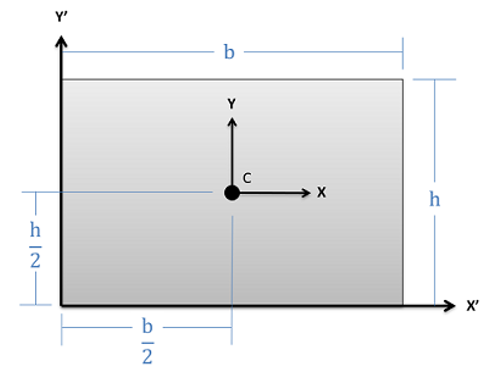$Area = bh$   $r_{cm} = \left[\frac{b}{2}, \frac{h}{2}\right]$ Right Triangle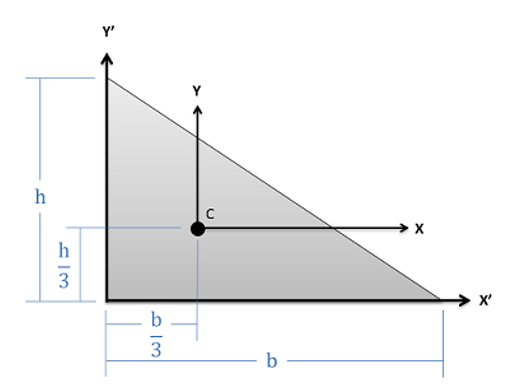$Area = \frac{1}{2}bh$   $r_{cm} = \left[\frac{b}{3}, \frac{h}{3}\right]$ Triangle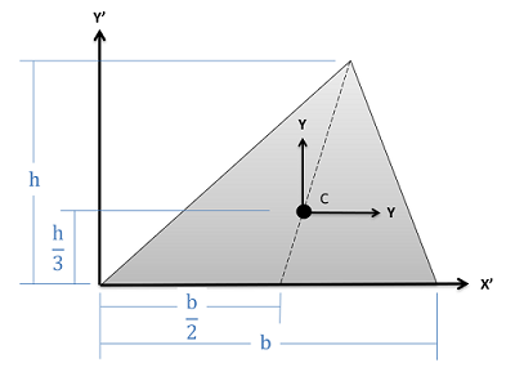$Area = \frac{1}{2}bh$   $r_{cm} = \left[\frac{b}{2}, \frac{h}{3}\right]$ Circle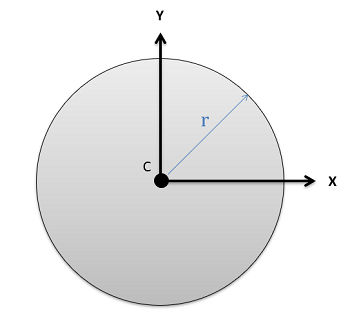$Area = \pi r^2$   $r_{cm} = \left[0,0\right]$ Circular Annulus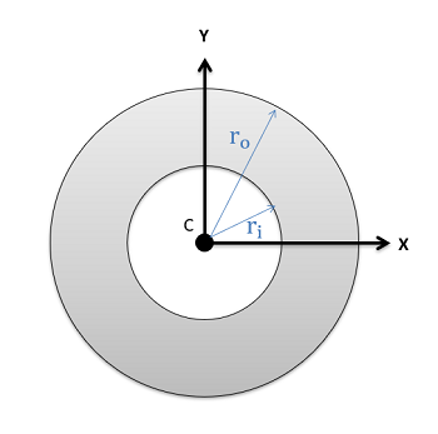$Area = \pi (r_o^2-r_i^2) \\r_o=\text{outer radius}\\r_i=\text{inner radius}$   $r_{cm} = \left[0,0\right]$ Semicircle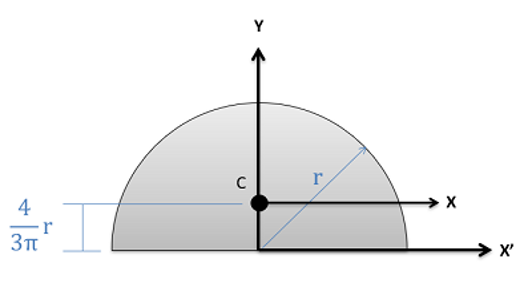$Area = \frac{\pi}{2}r^2$   $r_{cm} = \left[0,\frac{4}{3\pi}r\right]$ Quarter Circle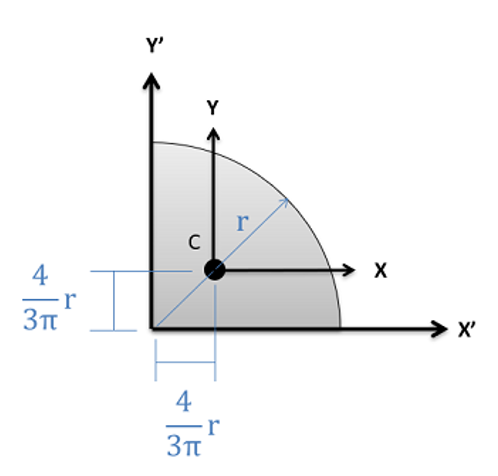$Area = \frac{\pi}{4}r^2$   $r_{cm}=\left[\frac{4}{3\pi}r,\frac{4}{3\pi}r\right]$ Ellipse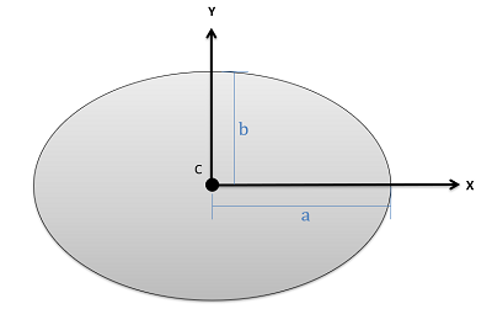$Area = \pi a b$   $r_{cm} = \left[0,0\right]$

#### Example 1: A Single Object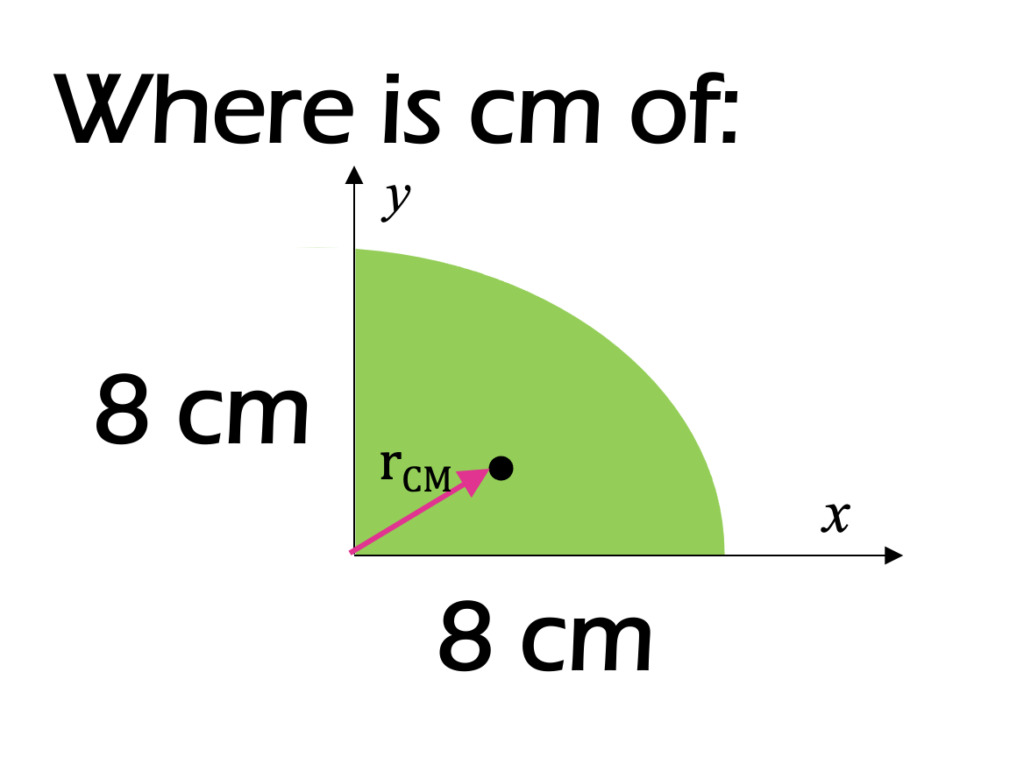To find the cm, select the appropriate shape from the above table.

.

.

.

.

.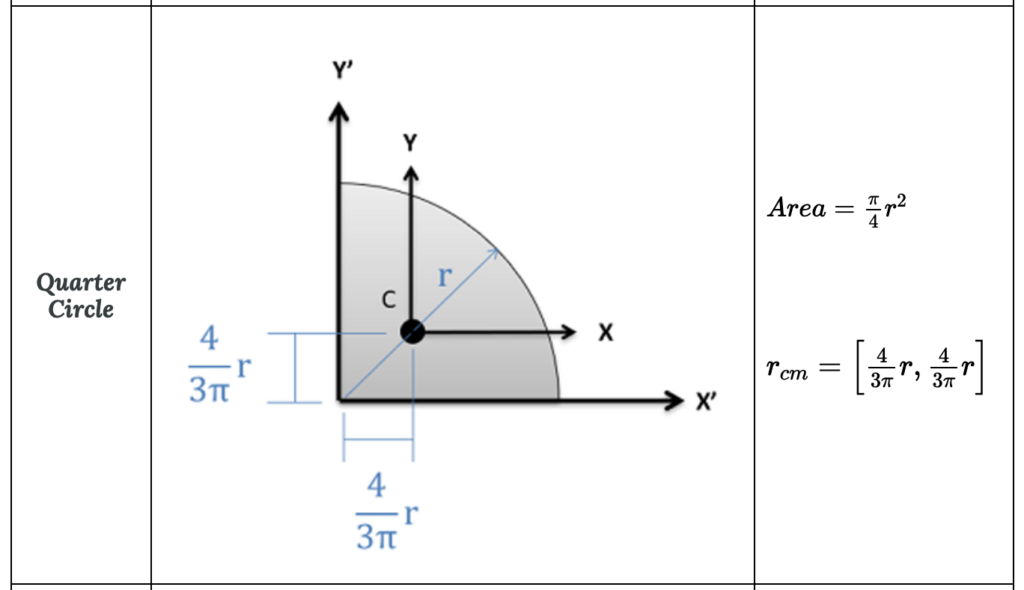Use the equation to solve for rcm.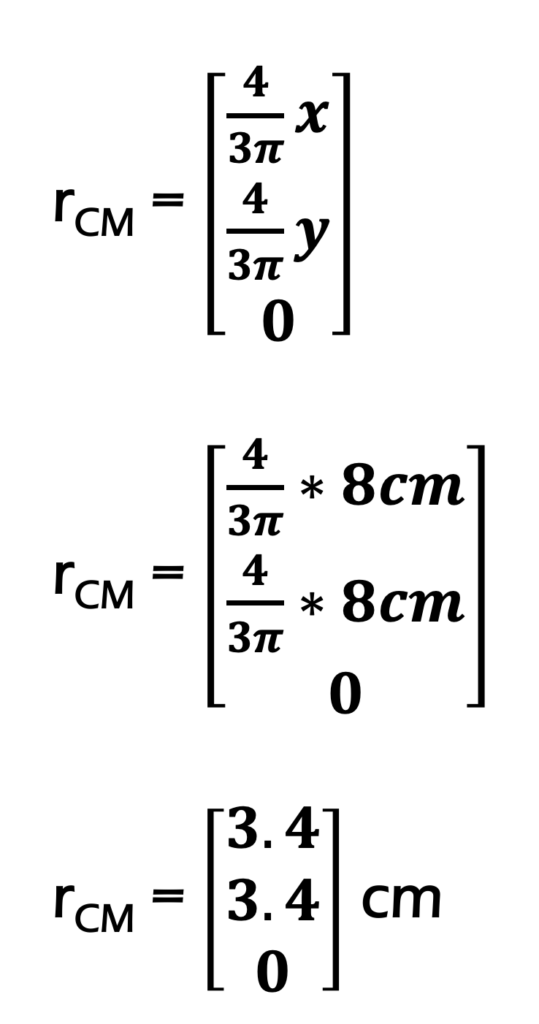This is an appropriate answer as it is less than the midpoint, where it would be for a square:

$\underline{\vec r}_{cm} = [3.4cm, 3.4cm, 0]$.

# 7.2.2 Composite Shapes

To find the center of mass of an object, you:

1. Define an origin
2. Split the object up into recognizable shapes
3. Find the center of mass (cm) of each shape from the origin
4. Calculate the mass of each part: $\rho = \frac{m}{V}$ (To find the centroid, this step can be skipped and only the area or volume is used).
5. Use the weighted cm equations to find the x cm. Repeat for y and z.

$$\bar x=\frac{\sum m_i x_i}{\sum m_i} \qquad \bar y=\frac{\sum m_i y_i}{\sum m_i} \qquad \bar z=\frac{\sum m_i z_i}{\sum m_i}$$

Start the process by labeling an origin point and axes on your shape. It will be important to measure all locations from the same point. Next, we must break our complex shape down into several simpler shapes. This may include areas or volumes (which we will count as positive areas or volumes) or holes (which we will count as negative areas or volumes). Each of these shapes will have a centroid (𝐶) or center of mass (𝐺) listed on the diagram.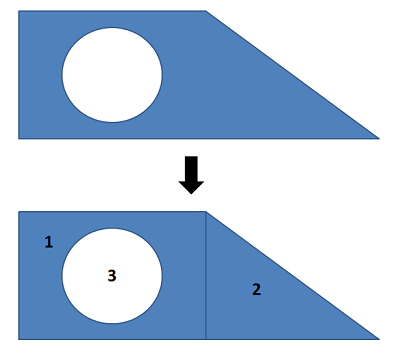For the shape shown at the top, we can break it down into a rectangle (1), a right triangle (2), and a circular hole (3). Each of these simple shapes is something we have listed in the centroid table to the right.

Once we have identified the different parts, we will create a table listing the area or volume of each piece, and the 𝑥 and 𝑦 centroid coordinates (or 𝑥, 𝑦, and 𝑧 coordinates in 3D). It is important to remember that each coordinate you list should be relative to the same base origin point that you drew in earlier. You may need to mentally adjust diagrams in the centroid tables so that the shape is oriented in the right direction, and account for the placement of the shape relative to the axes in your diagram.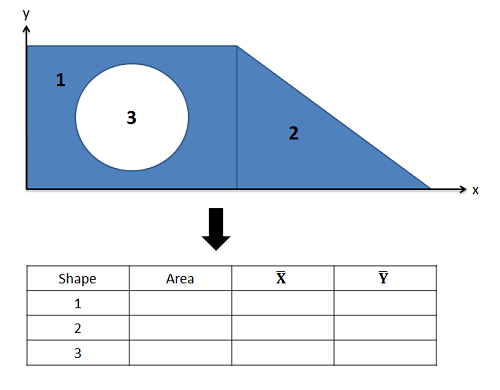For each of the shapes, we need to find the area and the x and y coordinates of the centroid. Remember to find the centroid coordinates relative to a single set of axes that is the same for all shapes.

Once you have the areas and centroid coordinates for each shape relative to your origin point, you can find the 𝑥 and 𝑦coordinate of the centroid for the overall shape with the following formulas. Remember that areas or volumes for any shape that is a hole or cutout in the design will be a negative area in your formula.

$$\bar x=\frac{\sum_{i=1}^n m_i x_i}{\sum_{i=1}^nm_i} \qquad \bar y=\frac{\sum_{i=1}^n m_i y_i}{\sum_{i=1}^nm_i}$$

This generalized formula to find the centroid’s 𝑥-location is simply Area 1 times $\bar x_1$ plus Area 2 times $\bar x_2$, plus Area 3 times $\bar x_3$, adding up as many shapes as you have in this fashion and then dividing by the overall area of your combined shape. The equations are the same for the 𝑦-location of the overall centroid, except you will instead be using $\bar y$-values in your equations.

For centroids in three dimensions we will simply use volumes in place of areas, and we will have a 𝑧 coordinate for our centroid as well as the 𝑥 and 𝑦 coordinates.

#### Example 2 – A Composite Object

For the following C shape, where is the center of mass?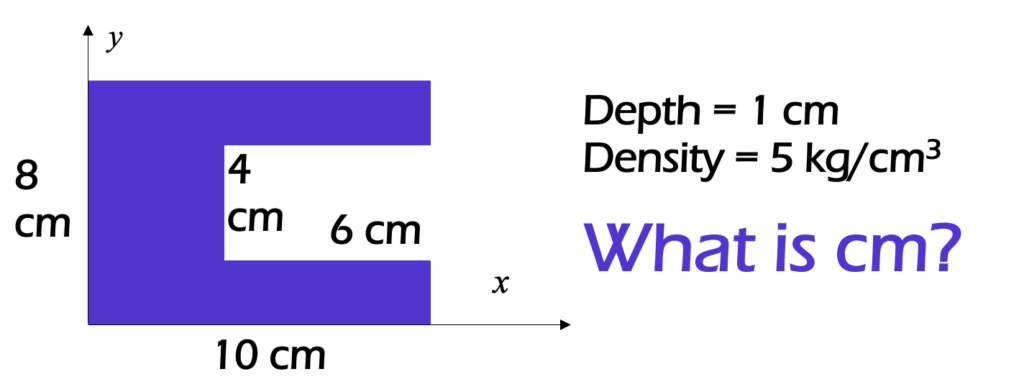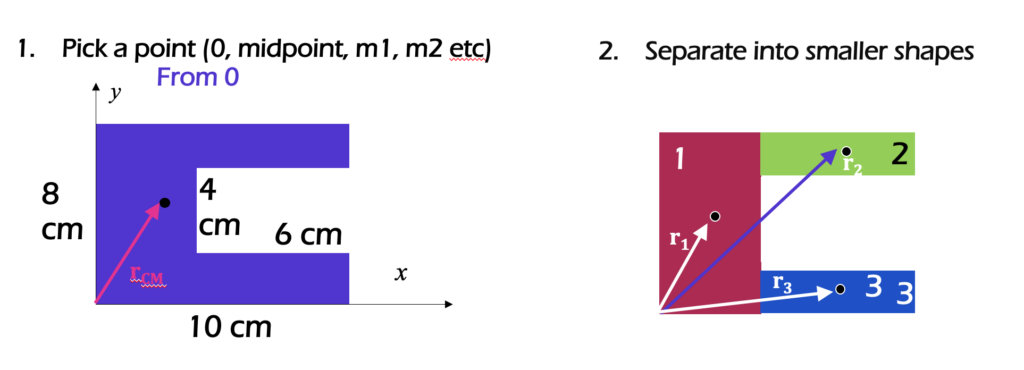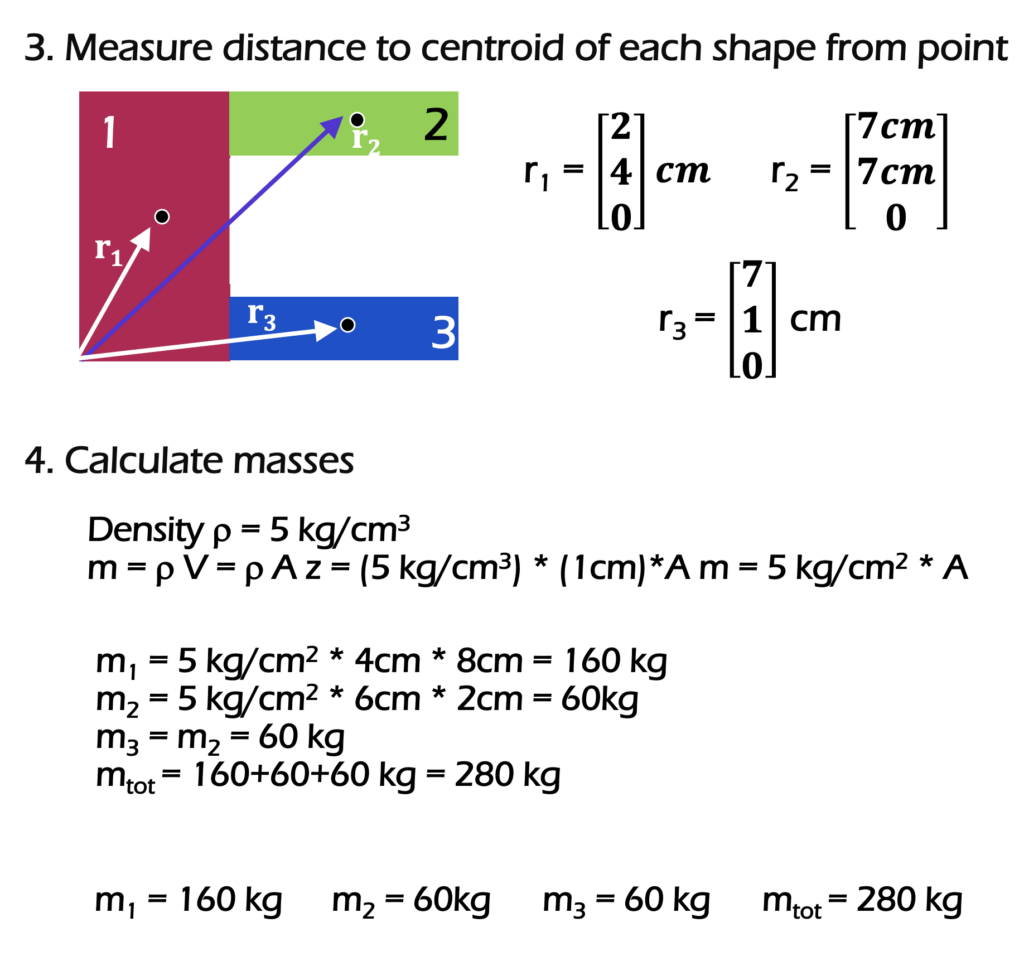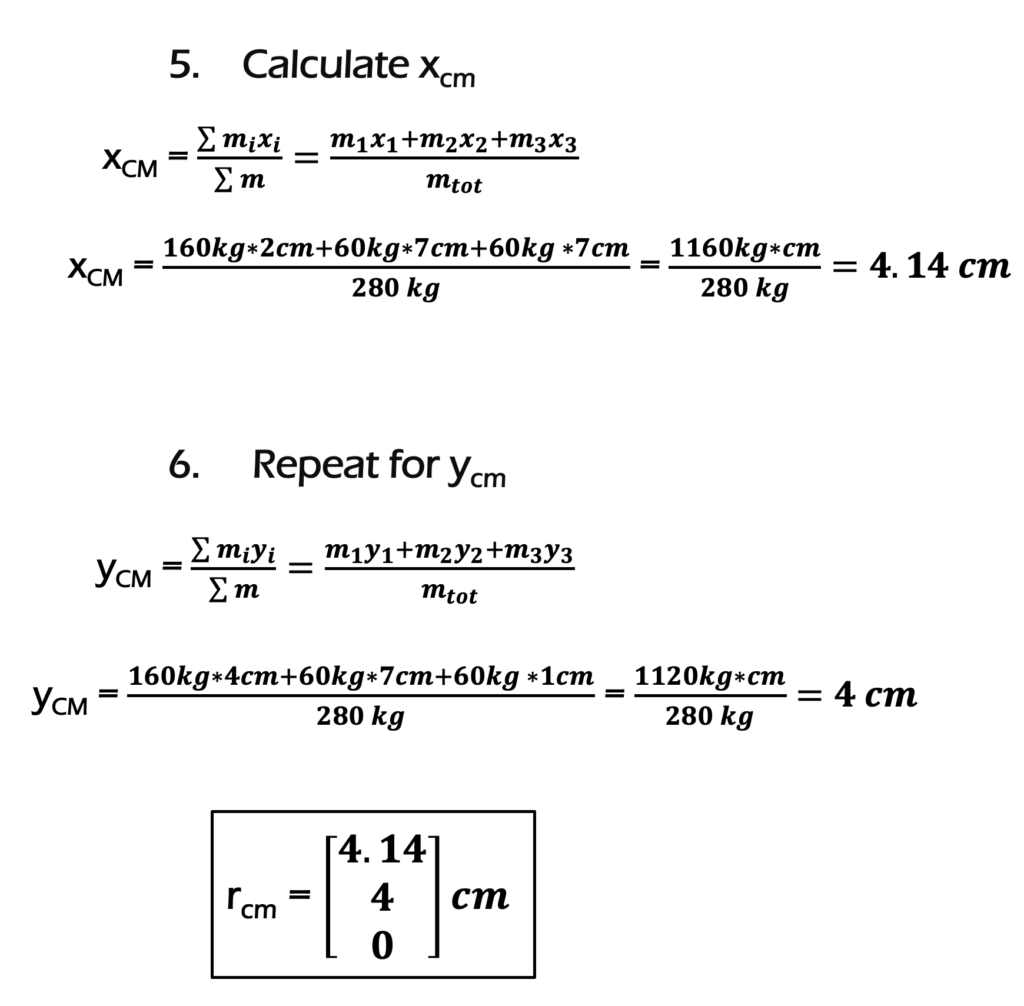This example used the density to calculate mass. To find the centroid, the area could have been used instead of the mass. However to find the center of mass, the density was used to calculate the mass of each object individually: $\rho = \frac{m}{V}$.

For the following C shape, where is the center of mass? (Use different shapes than above).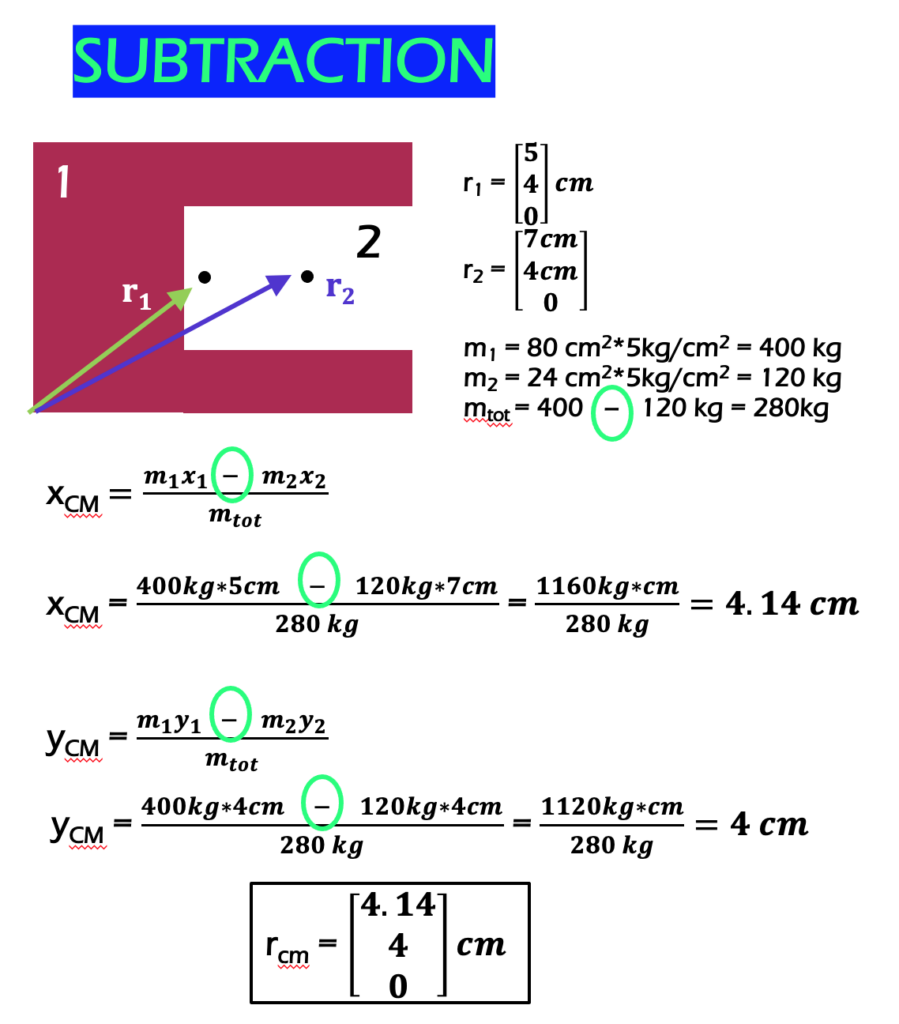The same answer is reached! This method involved only 2 shapes instead of 3.

There are more examples at http://mechanicsmap.psu.edu/websites/A2_moment_intergrals/method_of_composite_parts/methodofcompositeparts.html with pdfs and video solutions.

Key Takeaways

Basically: When there are complicated shapes, the center of mass can be found by breaking the shape up into better known shapes.

Application: To calculate the inertia of an object rotating about its center of mass, you will need to know where the center of mass is.

Looking Ahead: The next section will look at types of inertia.